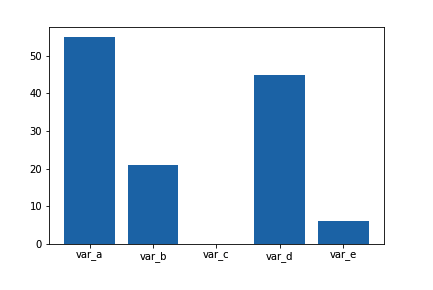Python:Matplotlib 和 Seaborn (二十五)

条形图

sb.countplot(data = df, x = 'cat_var')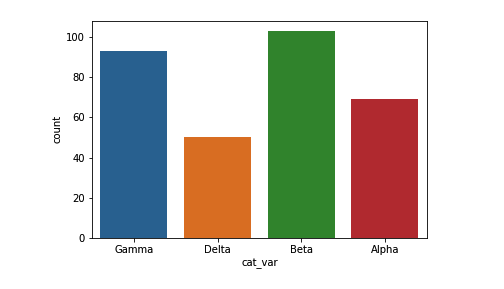base_color = sb.color_palette()
sb.countplot(data = df, x = 'cat_var', color = base_color)

color_palette 返回一个 RGB 元组列表，每个元组指定一个颜色。数字参数返回当前/默认调色板，我们将第一个颜色设为所有长条的颜色。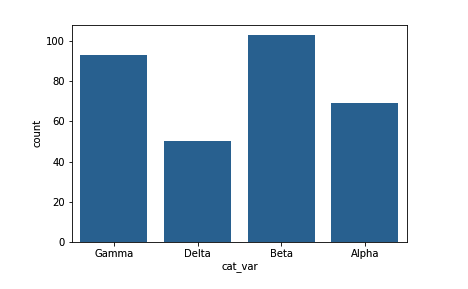base_color = sb.color_palette()
cat_order = df['cat_var'].value_counts().index
sb.countplot(data = df, x = 'cat_var', color = base_color, order = cat_order)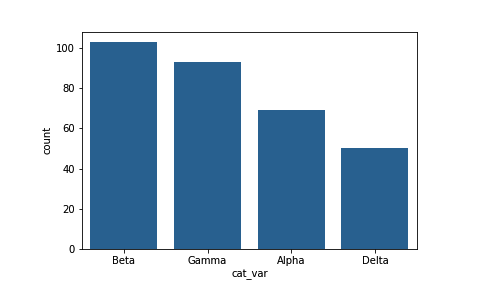# 本方法要求 pandas 0.21 或更高版本
level_order = ['Alpha', 'Beta', 'Gamma', 'Delta']
ordered_cat = pd.api.types.CategoricalDtype(ordered = True, categories = level_order)
df['cat_var'] = df['cat_var'].astype(ordered_cat)

## 如果你的 pandas 为 0.20.3 或更低版本，请使用这种方法：
# df['cat_var'] = df['cat_var'].astype('category', ordered = True,
#                                      categories = level_order)

base_color = sb.color_palette()
sb.countplot(data = df, x = 'cat_var', color = base_color)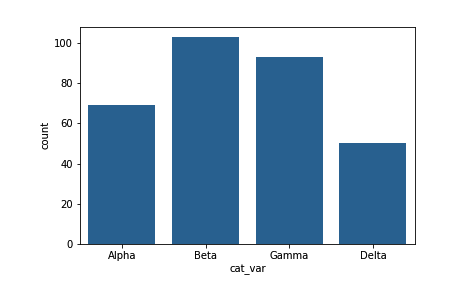其他变量

sb.countplot(data_var)

base_color = sb.color_palette()
sb.countplot(data = df, y = 'cat_var', color = base_color)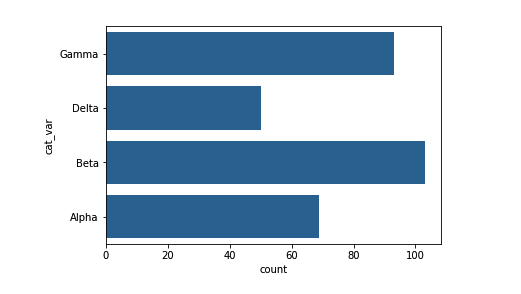base_color = sb.color_palette()
sb.countplot(data = df, x = 'cat_var', color = base_color)
plt.xticks(rotation = 90)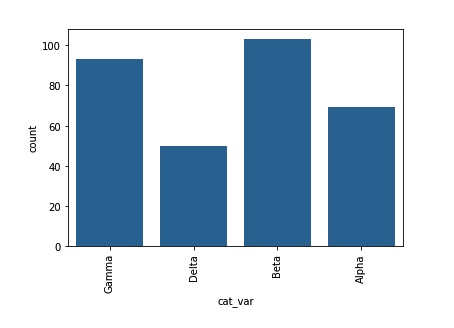绝对频率和相对频率

# get proportion taken by most common group for derivation
# of tick marks
n_points = df.shape
max_count = df['cat_var'].value_counts().max()
max_prop = max_count / n_points

# generate tick mark locations and names
tick_props = np.arange(0, max_prop, 0.05)
tick_names = ['{:0.2f}'.format(v) for v in tick_props]

# create the plot
base_color = sb.color_palette()
sb.countplot(data = df, x = 'cat_var', color = base_color)
plt.yticks(tick_props * n_points, tick_names)
plt.ylabel('proportion')

xticks 和 yticks 函数不仅仅会旋转刻度标签。你还可以获取和设置它们的位置及标签。第一个参数表示刻度位置：在此例中，刻度比例翻倍后变回计数比例。第二个参数表示刻度名称：在此例中，刻度比例的格式为精确到两位小数的字符串。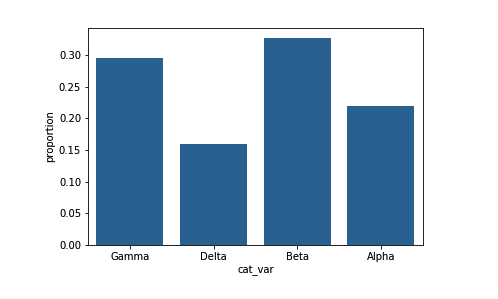其他版本

# create the plot
base_color = sb.color_palette()
sb.countplot(data = df, x = 'cat_var', color = base_color)

n_points = df.shape
cat_counts = df['cat_var'].value_counts()
locs, labels = plt.xticks() # get the current tick locations and labels

# loop through each pair of locations and labels
for loc, label in zip(locs, labels):

# get the text property for the label to get the correct count
count = cat_counts[label.get_text()]
pct_string = '{:0.1f}%'.format(100*count/n_points)

# print the annotation just below the top of the bar
plt.text(loc, count-8, pct_string, ha = 'center', color = 'w')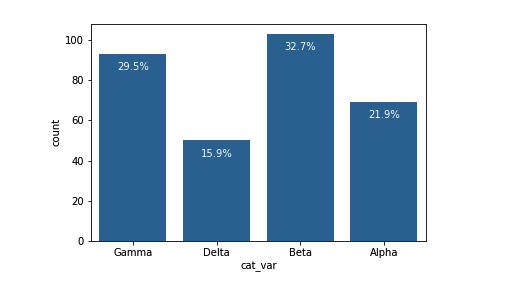计算缺失的数据

df.isna().sum()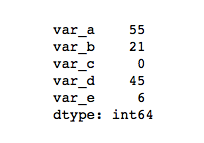na_counts = df.isna().sum()
base_color = sb.color_palette()
sb.barplot(na_counts.index.values, na_counts, color = base_color)